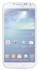#### TouchMaths_ME

The program TouchMaths (for touchscreen mobile phones) contain a set of revolutionary mathematical applications (Professional Calculator, Graph 2D, Function solver, Integral solver, Equation solver and Linear system...

Programming...

#### Calculator v.2

Calculator provides simple and advanced mathematical functions in a beautifully designed app. • Perform basic calculations such as addition, subtraction, multiplication, and division • Do scientific operations such as trigonometric, logarithmic, and exponential...